# Santos-Lopez de Haro hard sphere equation of state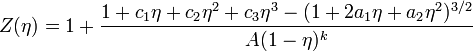$Z (\eta) = 1 + \frac{1+ c_1 \eta + c_2\eta^2 + c_3\eta^3 - (1 + 2a_1 \eta + a_2\eta^2)^{3/2}}{A(1-\eta)^k}$
where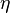$\eta$ is the packing fraction, given by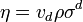$\eta = v_d \rho \sigma^d$,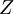$Z$ is the compressibility factor, and, for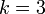$k=3$, one has (Table I):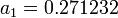$a_1= 0.271232$,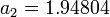$a_2= 1.94804$,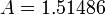$A=1.51486$,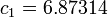$c_1=6.87314$,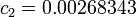$c_2=0.00268343$ and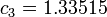$c_3= 1.33515$.